Editor Ratings:
User Ratings:
[Total: 0 Average: 0]

Linear program solver is a free software for Windows that solves mathematical linear programming problems using simplex method. In addition to linear programming, it also solves integer and goal programming problems. This software is capable of solving very large scale linear programming problems and that too very quickly.

Every student taking maths for higher studies has to go through Linear programming. And it takes quite some time to solve these problems. Linear Program solver comes in quite handy in solving these long problems in a few seconds. Its very helpful as it not only shows the results but also the process of solving the programming. So it can be used for leaning purposes also.

And since its a portable software so you can carry this free Linear Program solver in any external drive and use it on the go on any Windows PC without installing.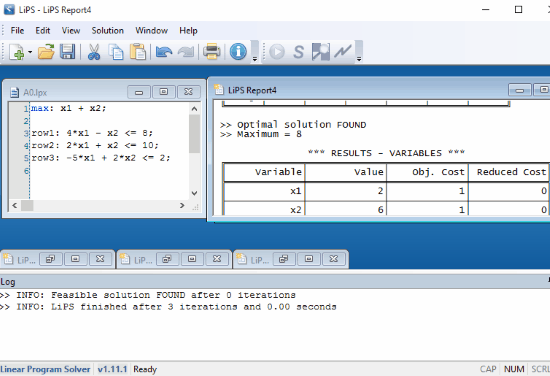I recently covered a very useful calculator to solve electrical equations having complex numbers, you can check it out if you want.

Now lets see how this works.

## How to Solve Linear Programming Using Linear program Solver:

Linear Program Solver is a portable software to solve Linear programming problems for Windows. After extracting the portable software from the downloaded ZIP file, you simply need to run the software without installing it. On running the software, its interface pops out with basic description of the software.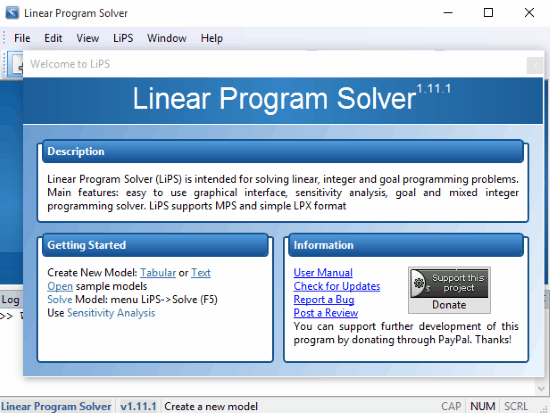Now coming to the software, you need to select Table Model from the dropdown of Create A New Model. Then fill the Number of Variables, Number of Constraints, and the Number of Objective according to the problem you are solving. Then select Maximization or Minimization according to your need and then press OK. On pressing OK, LiPS Model2 Window pops out where you need to fill the coefficients of different variables and coefficients present in the constraints.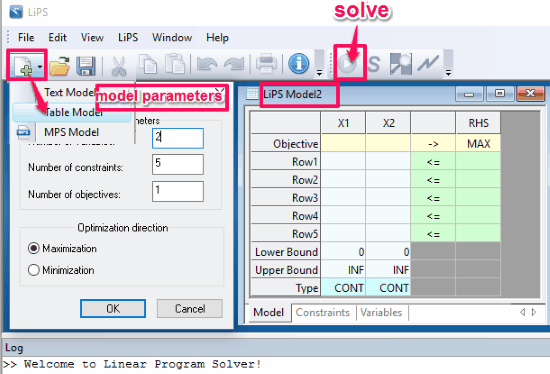After that you just need to select Solve option, and that’s how you get your solution.

Now let’s take the following example to see how it works (this example has been taken from phpsimplex.com).

Maximize Z = f(x,y) = 3x+2y

subject to:

2x + y <=18

2x + 3y<=42

3x + y<= 24

x>=0, y>=0

This problem has 2 variables, 5 constraints, and 1 objective to find the the maximum value of Z.

Now we need to fill Model Parameters first and then LiPS Model.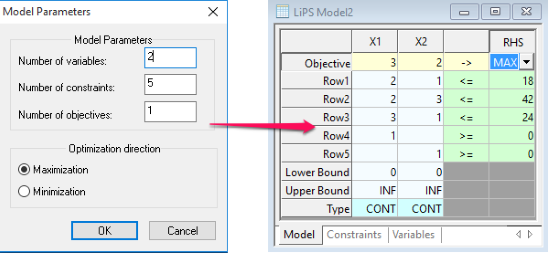On clicking on Solve option after filling above information, we get our solution.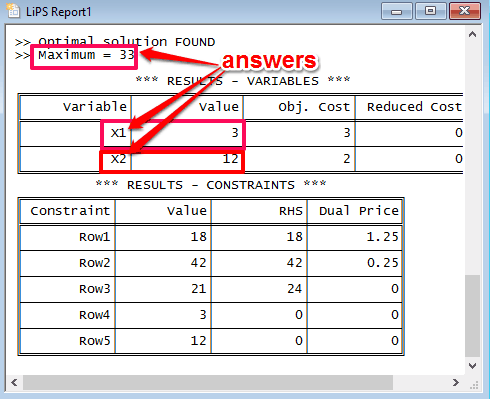So the result is Z maximum= 33, and the value of both the variables x1 and x2 is 3 and 12 respectively.

The whole process of solving this example can also be viewed in the software above the results, by scrolling up.

### Note:

The official website of this software says that it also solves integer programming and goal Programming, but I was not able to use these features, as these didn’t work for me. But you guys can try these features as well  and see if they work or not.

## Verdict:

This is a very good free Software for solving Linear programming by simplex method, and worked well for me during testing. And it really saves a lot of time in solving such problems. The icing on the cake is its very simple interface. So I would definitely recommend this to all the Maths lovers out there, to get your hand on this free software for solving your Linear programming problems in a few seconds.